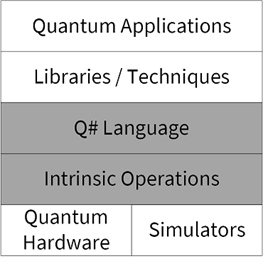# Chapter 7 What is a Quantum Algorithm?

### This chapter covers:

• What is a quantum algorithm?
• How to designoraclesto represent classical functions in quantum programs
• A first example of a quantum algorithm
• Several useful quantum programming techniques

One important application for quantum algorithms is in obtaining speedups for solving problems where we need to search over inputs to a function that we’re trying to learn about. Such functions could be obfuscated (such as hash functions), or could be computationally difficult to evaluate (common in studying mathematical problems). In either case, applying quantum computers to such problems requires us to understand how we program and provide input to quantum algorithms. To learn how to do so, we’ll program up and run an implementation of an algorithm known as the Deutsch–Jozsa algorithm, which will let us learn properties of unknown functions quickly using quantum devices.

##### Figure 7.1. In this Chapter, we will be covering topics in both the Q# language and intrinsic operations for quantum devices.## 7.1 CLASSICAL AND QUANTUM ALGORITHMS

 Algorithm noun: a step-by-step procedure for solving a problem or accomplishing some end. —Merriam–Webster Dictionary